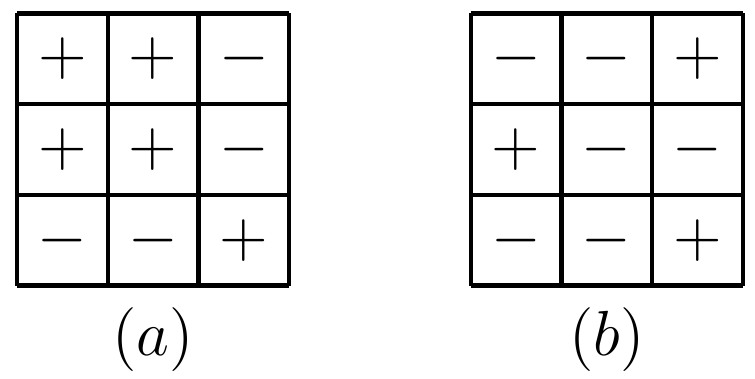###### back to index | new

Prove a positive proper fraction $\frac{m}{n}$ must be a sum of some reciprocals of distinct integers.

Let $p$ be an odd prime number. For positive integer $k$ satisfying $1\le k\le p-1$, the number of divisors of $k p+1$ between $k$ and $p$ exclusive is $a_k$. Find the value of $a_1+a_2+\ldots + a_{p-1}$.

Find all functions $f: \mathbb{R}\rightarrow \mathbb{R}$ such that $$f(yf(x)-x)=f(x)f(y)+2x$$ for all $x,\ y\in{\mathbb{R}}$.

Let $P(x)$ be a monic cubic polynomial. The lines $y = 0$ and $y = m$ intersect $P(x)$ at points $A$, $C$, $E$ and $B$, $D$, $F$ from left to right for a positive real number $m$. If $AB = \sqrt{7}$, $CD = \sqrt{15}$, and $EF = \sqrt{10}$, what is the value of $m$?

Show that $$x+n=\sqrt{n^2 + x\sqrt{n^2+(x+n)\sqrt{n^2+(x+2n)\sqrt{\cdots}}}}$$

Compute the value of $\sin 1^\circ \sin 2^\circ \cdots \sin 89^\circ$.

In $\triangle{ABC}$, $\angle{C}=\angle{A}+60^\circ$. If $BC=1$, $AC=r$ and $AB=r^2$, where $r > 1$, prove $r \le\sqrt{2}$.

Let $x$, $y$, $z$ be three positive real numbers satisfying $xyz+x+z=y$. Find the maximum value of $$P=\frac{2}{x^2 + 1}-\frac{2}{y^2+1}+\frac{3}{z^2+1}$$

Given any five real numbers, show that at least two of them $x$ and $y$ satisfy the condition $|xy+1|>|x-y|$.

Let $\{x_n\}$ and $\{y_n\}$ be two real number sequences which are defined as follow: $$x_1=y_1=\sqrt{3},\quad x_{n+1}=x_n +\sqrt{1+x_n^2},\quad y_{n+1}=\frac{y_n}{1+\sqrt{1+y_n^2}}$$ for all $n\ge 1$. Prove that $2 < x_ny_n < 3$ for all $n>1$.

Let $m$ be a positive integer. Show that $$\sin\frac{2}{\sqrt{m}}< \frac{2}{\sqrt{m+1}}$$

Prove $$\frac{1}{\sqrt{2019}} < \underbrace{\sin\sin\sin\cdots\sin}_{2017}\frac{\sqrt{2}}{2} < \frac{2}{\sqrt{2019}}$$

Let $a$ and $b$ be two positive real numbers not exceeding $1$. Prove $$\frac{1}{\sqrt{a^2 + 1}}+\frac{1}{\sqrt{b^2 +1}}\le\frac{2}{\sqrt{1+ab}}$$

$\textbf{Flip the Grid}$

Given two grids shown below, is it possible to transform ($a$) to ($b$) after a series of operations? In each operation, one can change all the signs in either one entire row or one entire column.Is it possible to use twenty seven $1\times 2\times 4$ blocks to construct a $6\times 6\times 6$ cube?

Find all pairs of positive integers $(a, b)$ satisfying $a! + b! = a^b + b^a$.

Let $f$ be a function from $\mathbb{N}$ to $\mathbb{N}$ such that

(i) $f(1)=0$

(ii) $f(2n)=2f(n)+1)$

(iii) $f(2x+1)=2f(n)$

Find the least value of $n$ such that $f(n)=2016$.

Let $(x^{2017}+x^{2019}+2)^{2018} = a_0+a_1x+\cdots+a_nx^n$. Find $$a_0-\frac{a_1}{2}-\frac{a_2}{2}+a_3-\frac{a_4}{2}-\frac{a_5}{2}+a_6-\cdots$$

Find all polynomials with integer coefficients $p(x)$ that satisfy the following identity $$2p(2x)=p(3x)+p(x)$$.

Find the number of non-decrease sequences of length $n$ and each element is a non-negative integer not exceeding $d$.

Find, with proof, all pairs of positive integers $(n, d)$ with the following property: for every integer $S$, there exists a unique non-decreasing sequence of n integers $a_1$, $a_2$, $\cdots$, $a_n$ such that $a_1 + a_2 + \cdots + a_n = S$ and $a_n-a_1 = d$.

Compute $\underbrace{3^{3^{3^{\cdots^{3}}}}}_{2012\ times}\pmod{100}$.

Show that $x^5\equiv 3\pmod{11}$ is not solvable.

Find one solution to $x^7\equiv 3\pmod{11}$.

How many positive integers not exceeding $100$ are there such that the value of $(3^x-x^2)$ is a multiple of $5$?